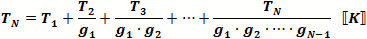# Cascaded Noise Figure & Noise Temperature

Noise figure (NF) is the increase in noise power of a device from the input to the output that is greater than the signal gain. In effect, it is the amount of decrease of the signal-to-noise ratio. Like gain, noise figure can be expressed either as a ratio or in decibels (dB). Note: NF is not a power level, so it never has units of dBm.

As the following equation shows, cascaded noise figure is affected most profoundly by the noise figure of components closest to the input of the system as long as some positive gain exists in the cascade. If only loss exists in the cascade, then the cascaded noise figure equals the magnitude of the total loss.

Cascading Noise Figure Values in a Chain of ComponentsClick here to view an example of a cascaded system.
Calculating the cascaded values for noise figure (NF) for the system budget requires the following operation based upon ratios for gain and noise figure (do not use decibel values). The standard format for indicating decibel values is to use upper case letters, i.e., G and NF for units of dB; the standard format for indicating ratio values is to use lower case letters, i.e., g and nf.

Conversions:   nf = 10NF/10  ↔  NF (dB) = 10 * log10 (nf)

where nf is a unitless ratio and NF has units of dBA Typical Chain of Cascaded ComponentsCombining 2 Stages at a Time for Calculations See cascade calculations for NF, IP2, IP3, and P1dB.

This equation gives the method for calculating cascaded NF (nf) values based on the nf and gain of each stage. When using the formula in a software program or in a spreadsheet, it is more convenient and efficient to calculate each successive cascaded stage with the one preceding it using the following format, per the drawing (above-right).Click here to see Agilent's App Note 1303 on using a spectrum analyzer to measure noise figure.

Conversion Between Noise Figure and Noise Temperature

Noise Temperature (K) =Noise Figure (dB) =These equations assume a room temperature reference (290 K). If applicable, use whatever your reference temperature is in place of the 290K shown.About RF CafeCopyright: 1996 - 2024Webmaster:    Kirt Blattenberger,    BSEE - KB3UON RF Cafe began life in 1996 as "RF Tools" in an AOL screen name web space totaling 2 MB. Its primary purpose was to provide me with ready access to commonly needed formulas and reference material while performing my work as an RF system and circuit design engineer. The World Wide Web (Internet) was largely an unknown entity at the time and bandwidth was a scarce commodity. Dial-up modems blazed along at 14.4 kbps while typing up your telephone line, and a nice lady's voice announced "You've Got Mail" when a new message arrived... All trademarks, copyrights, patents, and other rights of ownership to images and text used on the RF Cafe website are hereby acknowledged. My Hobby Website:  AirplanesAndRockets.comPlease Support RF Cafe by purchasing my  ridiculously low−priced products, all of which I created. These Are Available for Free